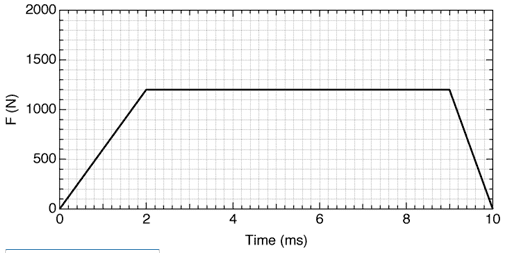# Force and Impulse

#### huybinhs

1. The problem statement, all variables and given/known data

The Figure shows an approximate representation of the contact force versus time during the collision of a 36-g tennis ball with a wall. The initial velocity of the ball is 151.6 m/s perpendicular to the wall. What is the speed of the tennis ball after the collision?2. Relevant equations

P = mv

3. The attempt at a solution

I did find 3 Area in the pic the add them up. I got 10200 total, then what's next?
Any help would be appreciated! ;)

Gold Member
P0 + J = Pf

#### huybinhs

P(0) = (36/1000) * 151.6 = 5.4576 N*m

Pf = (36/1000) * vf with Pf = total of 3 Areas, correct?

#### thrill3rnit3

Gold Member
P(0) = (36/1000) * 151.6 = 5.4576 N*m

Pf = (36/1000) * vf with Pf = total of 3 Areas, correct?
No, in the equation I told you, J represents the area of the figure. Do you see why?

#### huybinhs

so P(0) = (36/1000) * 151.6 = 5.4576 N*m

then 5.4576 + 10200 = (36/1000) vf => vf = 2.83 * 10^5 m/s, right?

#### thrill3rnit3

Gold Member
so P(0) = (36/1000) * 151.6 = 5.4576 N*m

then 5.4576 + 10200 = (36/1000) vf => vf = 2.83 * 10^5 m/s, right?
Time in the graph is given in milliseconds (ms).

#### huybinhs

If so 10200 will be = 10.2

=> 5.4576 + 10.2 = (36/1000) vf => vf = 435 m/s Wrong!!! Why?

#### thrill3rnit3

Gold Member
If so 10200 will be = 10.2

=> 5.4576 + 10.2 = (36/1000) vf => vf = 435 m/s Wrong!!! Why?
Does the force act on the same direction as the motion?

#### huybinhs

so u mean J in this case is just = 9 ?

#### thrill3rnit3

Gold Member
so u mean J in this case is just = 9 ?
I mean it's negative, and where did you get 9?

#### huybinhs

so I got the 1st area = 0.5 * 0.002 * 1200 = 1.2

2nd = 0.007 * 1200 = 8.4

3rd = 0.001 * 0.5 * 1200 = 0.6

so now why should i do? plz be more specific!

#### thrill3rnit3

Gold Member
so I got the 1st area = 0.5 * 0.002 * 1200 = 1.2

2nd = 0.007 * 1200 = 8.4

3rd = 0.001 * 0.5 * 1200 = 0.6

so now why should i do? plz be more specific!
add the 3 = 10.2

I'm saying that since the force of the wall is acting opposite of the motion, then maybe the impulse should be negative (-10.2)

#### huybinhs

Got it! Thanks for your patience ;)

#### thrill3rnit3

Gold Member
Got it! Thanks for your patience ;)
You're most welcome### The Physics Forums Way

We Value Quality
• Topics based on mainstream science
• Proper English grammar and spelling
We Value Civility
• Positive and compassionate attitudes
• Patience while debating
We Value Productivity
• Disciplined to remain on-topic
• Recognition of own weaknesses
• Solo and co-op problem solving